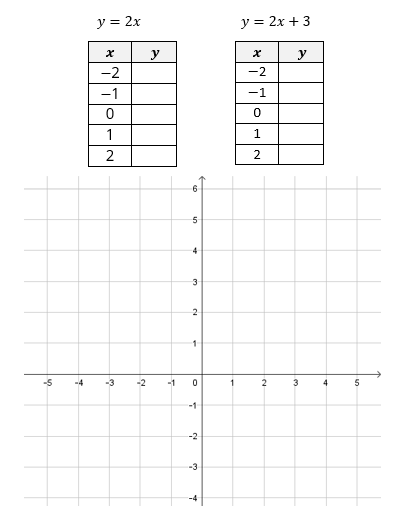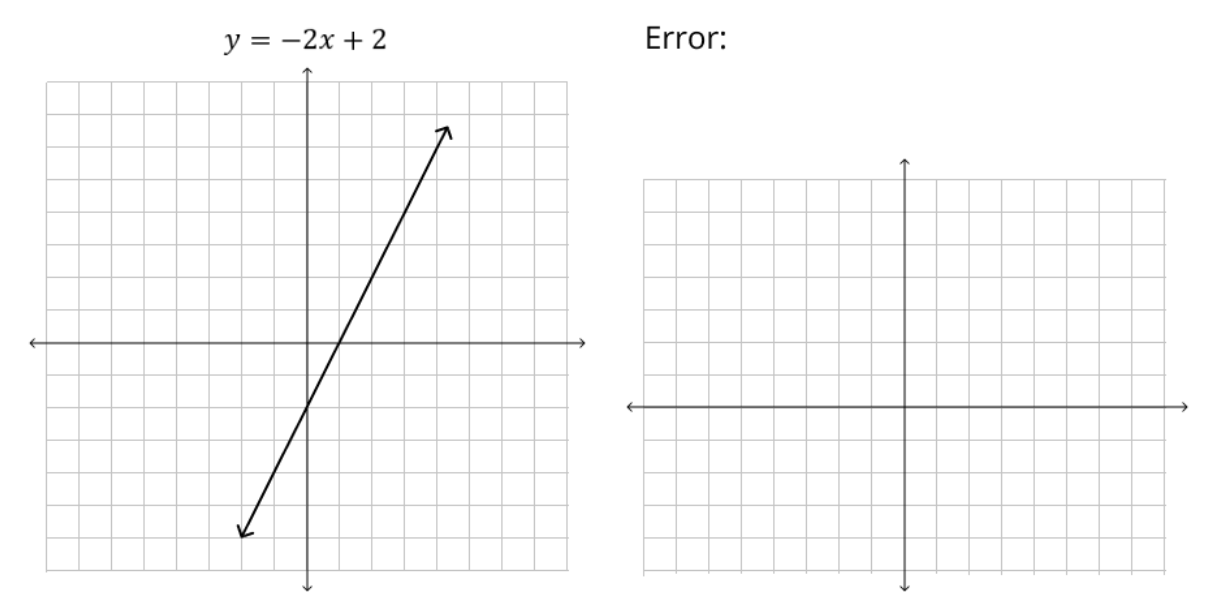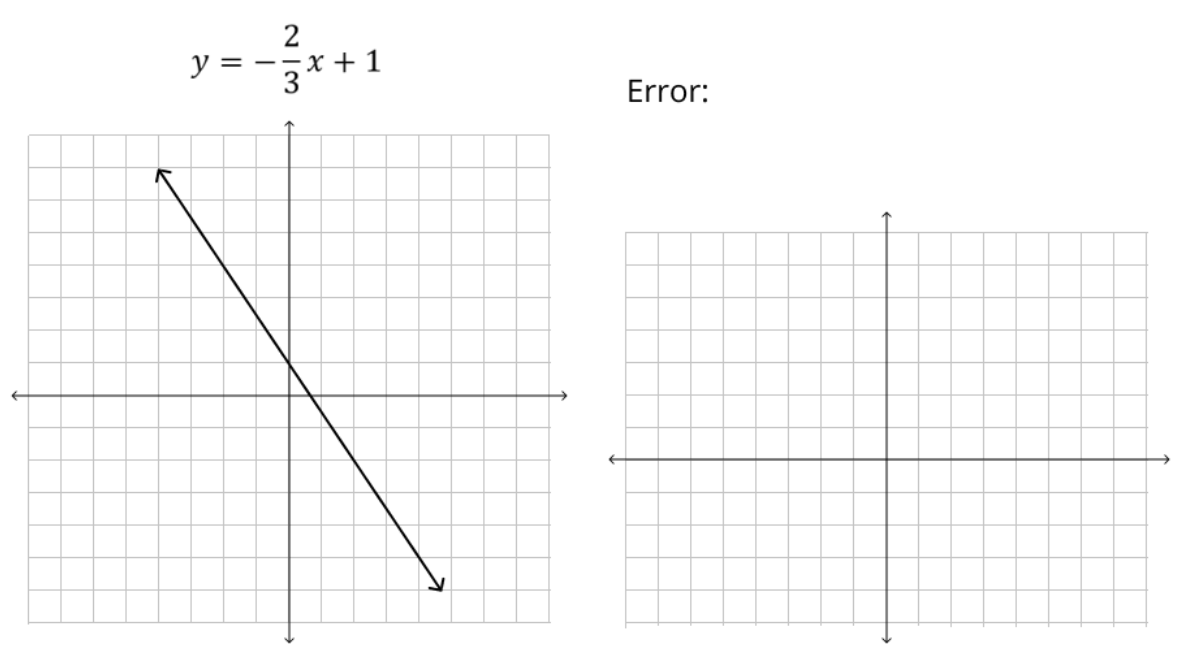# Linear Relationships

## Objective

Graph linear equations using slope-intercept form ${y = mx + b}$.

## Common Core Standards

### Core Standards

?

• 8.EE.B.6 — Use similar triangles to explain why the slope m is the same between any two distinct points on a non-vertical line in the coordinate plane; derive the equation y = mx for a line through the origin and the equation y = mx + b for a line intercepting the vertical axis at b.

• 8.F.A.3 — Interpret the equation y = mx + b as defining a linear function, whose graph is a straight line; give examples of functions that are not linear. For example, the function A = s² giving the area of a square as a function of its side length is not linear because its graph contains the points (1,1), (2,4) and (3,9), which are not on a straight line.

?

• 8.G.A.1

## Criteria for Success

?

1. Understand that the lines of equations ${y=mx}$ and ${y=mx}+b$ have the same slope $m$.
2. Understand that ${y=mx}+b$ is the translation of the line ${y=mx}$ by a vertical distance $b$
3. Define ${y=mx}+b$ as the slope-intercept form of a linear equation where $m$ represents slope and $b$ represents the value of the $y$-intercept
4. Identify the slope and $y$-intercept of an equation in form ${y=mx}+b$, and use them to draw the line that represents the equation.

## Tips for Teachers

?

• In Lesson 8, students are introduced to the slope-intercept form of a linear equation, and they see how it is derived from the proportional equation ${y=mx}$. They use this form as an efficient way to draw the graph of a linear equation. In Lesson 9, students will encounter equations in standard form, ${ax+by=c}$, and they will write these equations into slope-intercept form to graph them.

### Lesson Materials

• Patty paper (transparency paper) (1 sheet per student)
• Ruler (1 per student)

### Remote Learning Guidance

If you need to adapt or shorten this lesson for remote learning, we suggest prioritizing Anchor Problem 1 (benefits from discussion) and Anchor Problem 2 (benefits from worked example). Find more guidance on adapting our math curriculum for remote learning here.

#### Fishtank Plus

• Problem Set
• Student Handout Editor
• Vocabulary Package

## Anchor Problems

?

### Problem 1

Two linear equations are shown below. Complete the table of values for each one and graph the lines in the same coordinate plane. Then answer the questions that follow.1. ${y=2x}$ is a proportional relationship represented by a line through the origin (0, 0).  In ${y=2x}+3$, what impact does the “+3” have on the table of values? What impact does it have on the lines in the graph? What transformation is this?
2. What is the y-intercept of each line? Where do you see this in the equations?
3. What is the slope of each line? Where do you see this in the equations?
4. Describe the graph of the line ${y=2x}-3$.

### Problem 2

For each linear equation below, identify the slope and $y$-intercept and use them to graph the line.

1. $y={1\over3} x-2$
2. $y=-3x+4$
3. $y=-x$

## Problem Set

?The following resources include problems and activities aligned to the objective of the lesson that can be used to create your own problem set.

• Include problems where students match an equation to a graph by matching slope and $y$-intercepts.

a.b.